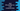# Java program to find the kth smallest number in an unsorted array## Java program to find the kth smallest number in an unsorted array :

This tutorial is to find the kth smallest element of an integer array ( unsorted ). To find kth smallest array, we can simply sort the array in increasing order and find out the kth positioned number.

Example :

``````Given array = [3,9,5,6,2,1]

Q: Find second smallest number

1. Sort the array
array = [1,2,3,5,6,9]

2. 2nd Smallest number is array[2-1] = array = 2

``````

For this approach, we need to sort the numbers first. We can use any type of sort we want. Like quick sort, selection sort etc. In java , if we will store the elements in an array, we can also use ‘Arrays.sort()’ to sort the elements.

### Second Approach : Min-Heap using Priority Queue :

Using priority queue, or min heap we can find out the kth smallest element. Following algorithm we are going to use in this program :

1. First create one Priority Queue

2. Insert all elements to this priority queue

3. Extract elements one by one from this priority queue. The kth element extracted is the kth smallest element

### Java Program :

``````import java.util.PriorityQueue;
import java.util.Random;
import java.util.Scanner;

public class Main {
/**
* Utility function to print
*/
private static void println(String str) {
System.out.println(str);
}

private static void print(String str) {
System.out.print(str);
}

/**
* Find the Kth smallest element of an array
*
* @param array : Given array
* @param k     : value of K
* @return : Kth smallest element
*/
public static int findKthSmallestElement(int[] array, int k) {

PriorityQueue priorityQueue = new PriorityQueue();

for (int i = 0; i < array.length; i++) { priorityQueue.offer(array[i]); } println("Final priority-queue " + priorityQueue); int currentNo = 0; while (k > 0) {
currentNo = priorityQueue.poll();
k--;
}

return currentNo;
}

/**
* Utility method to print all elements of an array
*
* @param arr : Array to print
*/
private static void printArray(int[] arr) {
for (int i = 0; i < arr.length; i++) {
print(arr[i] + " ");
}
}

public static void main(String args[]) {
Random random = new Random();

Scanner scanner = new Scanner(System.in);

println("How many numbers you want to add to the array : ");
int total_num = scanner.nextInt();

int[] num_array = new int[total_num];

for (int i = 0; i < total_num; i++)
num_array[i] = Math.abs(random.nextInt(10000));

println("Random array : ");
printArray(num_array);
println("");

println("Enter k : ");
int k = scanner.nextInt();

System.out.println("Smallest element for k = " + k + " : "
+ findKthSmallestElement(num_array, k));
}
}
``````

### Sample Output :

``````How many numbers you want to add to the array :
10
Random array :
4552 8757 4930 526 9889 4616 1114 7243 3910 5314
Enter k :
3
Final  priority-queue [526, 3910, 1114, 4552, 5314, 4930, 4616, 8757, 7243, 9889]
Smallest element for k = 3 : 3910
``````

### Explaination :

1. First , we take the number of elements from the user.

2. Using a ‘for’ loop, insert random numbers to an array. The random number is ranged between ‘0’ and ‘1000’

3. Take the value of ‘k’ from user

4. Now create one PriorityQueue and insert all numbers to it.

5. Extract all elements one by one for ‘k’ times. ‘k’th number is the required number.